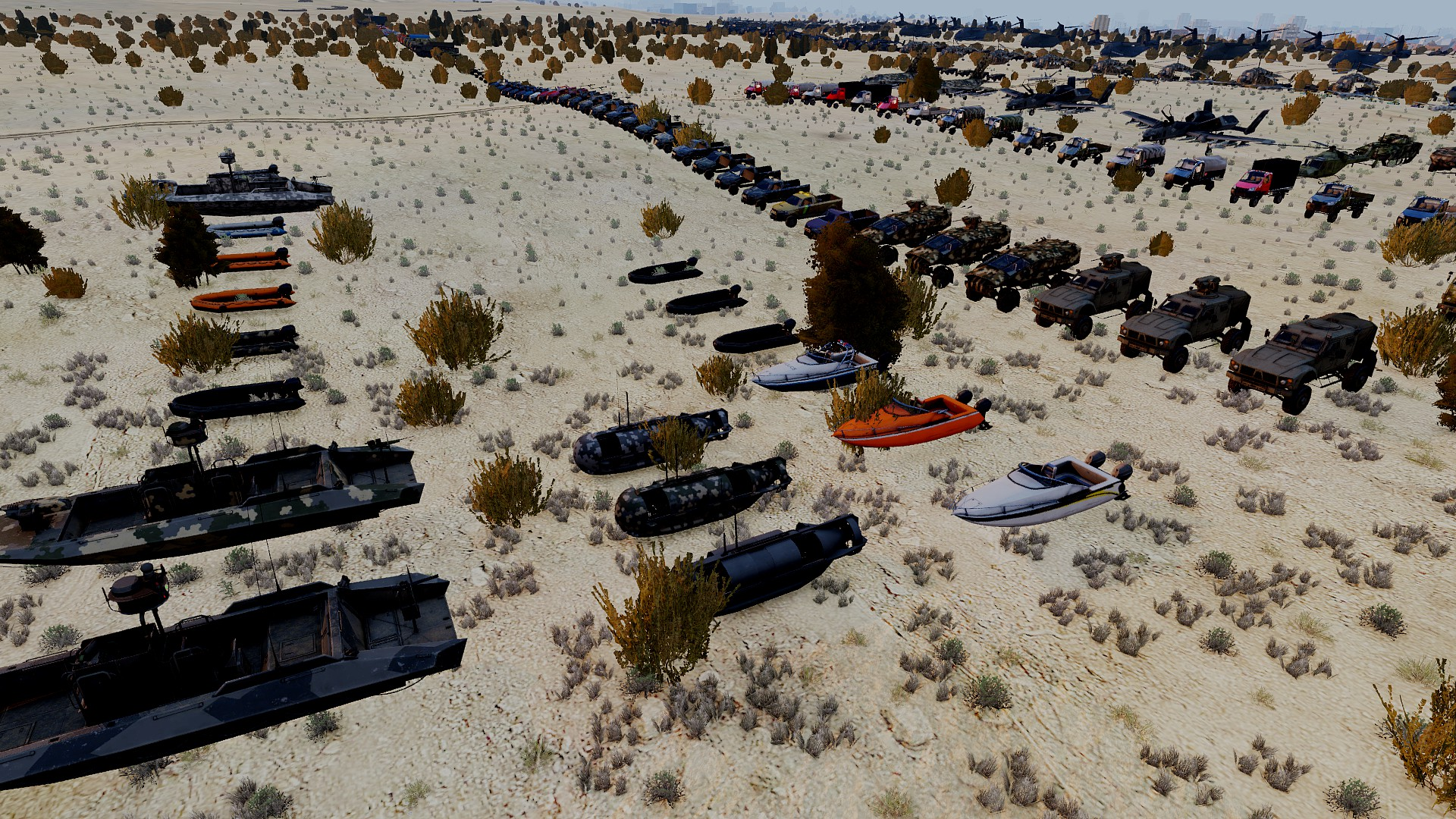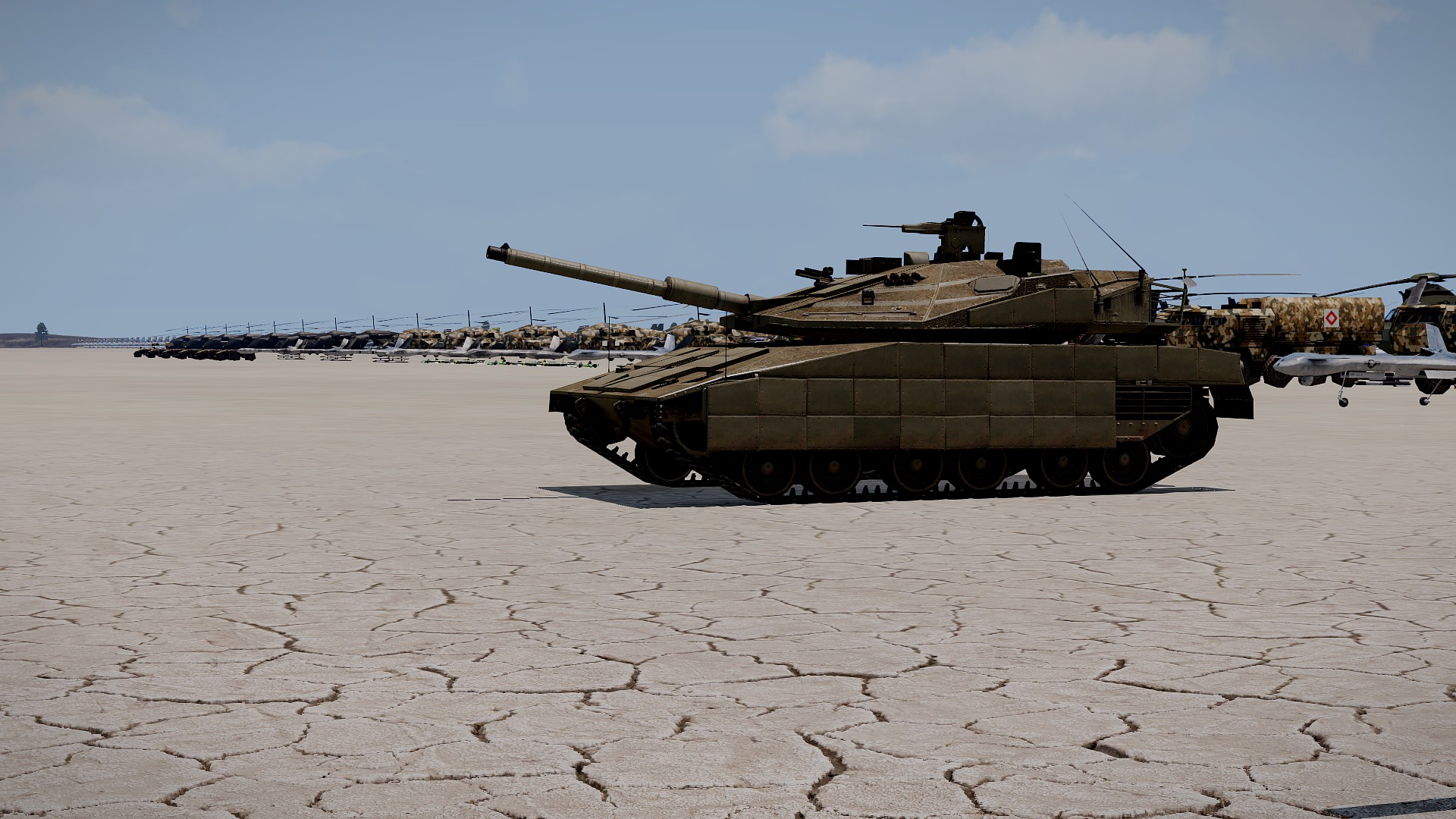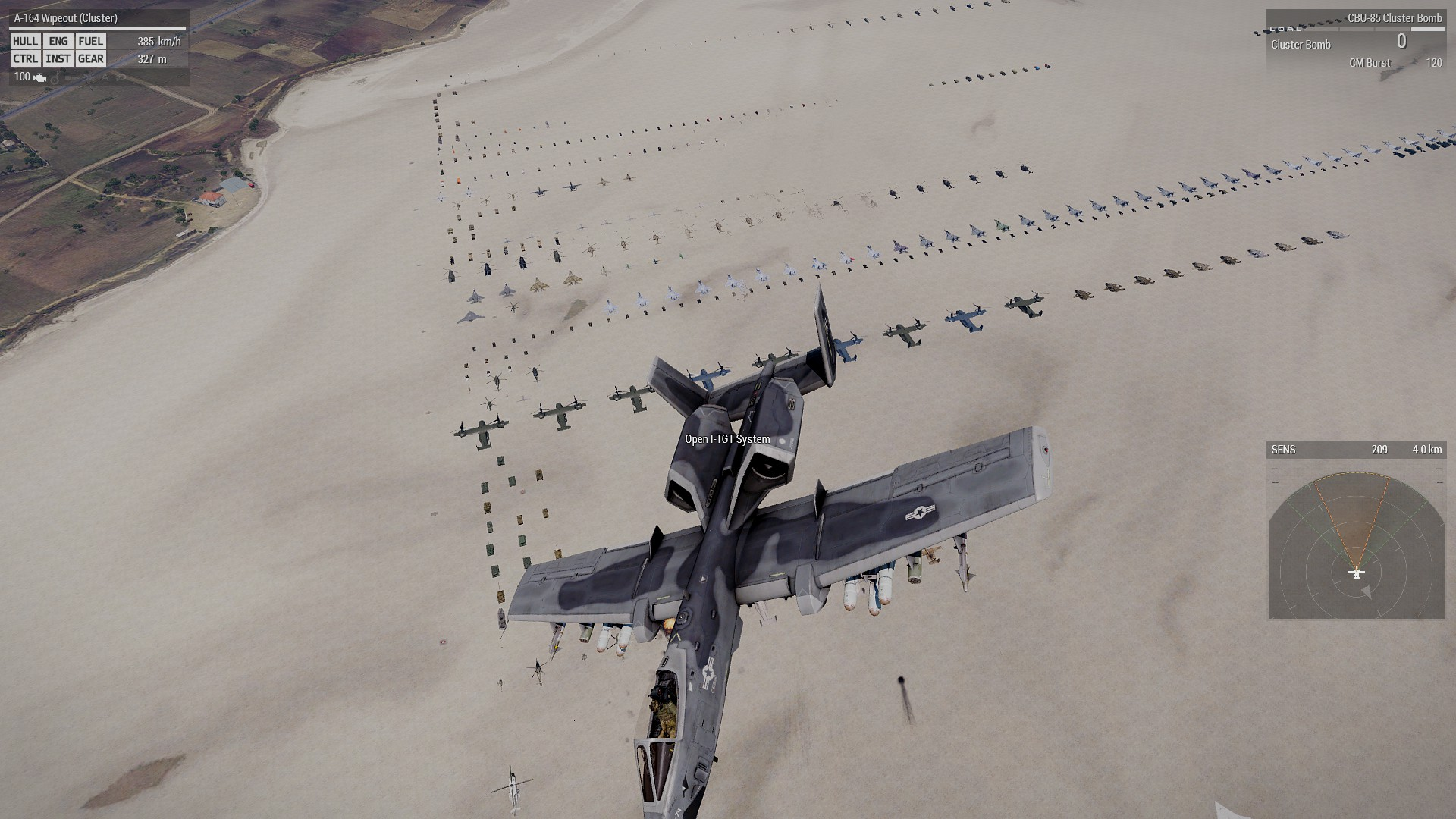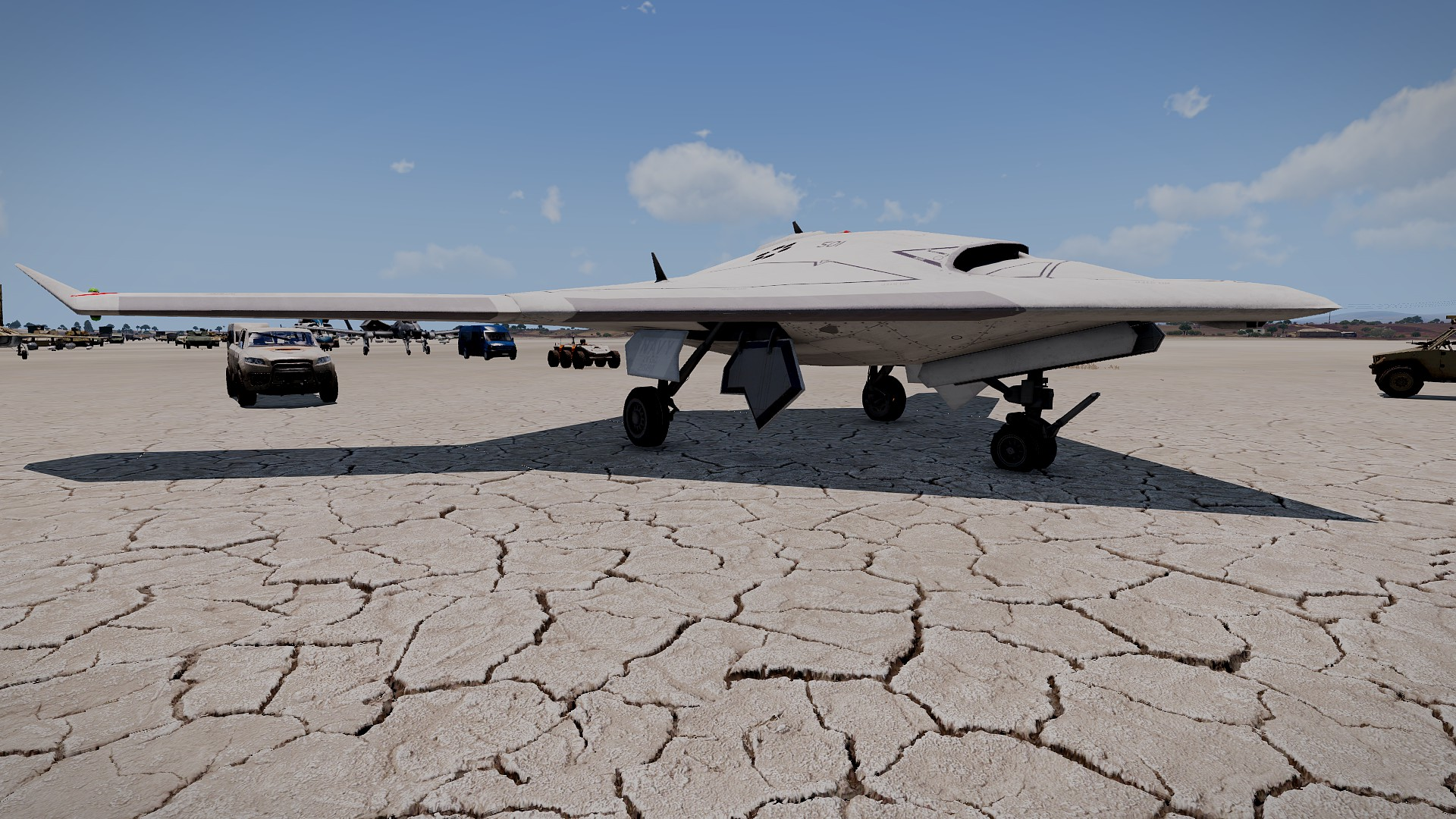## Recommended Posts

Just another topic... I am using loadFile to pull out a text file of an array I made for object creation and assortment.
However in the end it doesn't work out for me because I can't quite pull the text out of the array without getting a type error.
At least I tried....

I thought it was tricky enough pulling out the code and getting it into a character again, but I
pulled it off and got it working around.

I'm sure there's a better way of making objects, or classes...

Spoiler
```

private _contents = [] ;

//hint (str _contents) ;

_contentsAry = [] ;
_contentsAry = toArray _contents ;
//private _asChars = [] ;
//private _asChars = toString _contentsAry ;
_numCharsFile = count _contentsAry ;

private _spotInLine = [] ;

for [{ private  _zx = 0 }, { _zx < _numCharsFile }, { _zx = _zx + 1 }] do
{

private _spotInFile = ""  ;
_spotInFile = ( _contentsAry select _zx ) ;
_spotInLine pushBack _spotInFile ;

//hint (str _spotInFile ) ;

//sleep .03 ;

};

private _asWas = toString _spotInLine ;
hint (str _asWas ) ;
_numCharsFile2 = count _asWas ;
sleep 2 ;
hint (str "GO" ) ;
sleep 2 ;
for [{ private  _ux = 0 }, { _ux < _numCharsFile2 }, { _ux = _ux + 1 }] do
{

private _spotInFile2 = ""  ;
_spotInFile2 = ( _asWas select [_ux,1] ) ;
hint (str _spotInFile2 ) ;

sleep 2 ;
};

/*
spotAsNum = _spotInFile ;
_spotAsChar = (str _spotInFile );
*/
//deception... follow suite ... make money off of pesky worthless moderators... free speech is valuable

```

##### Share on other sites
Spoiler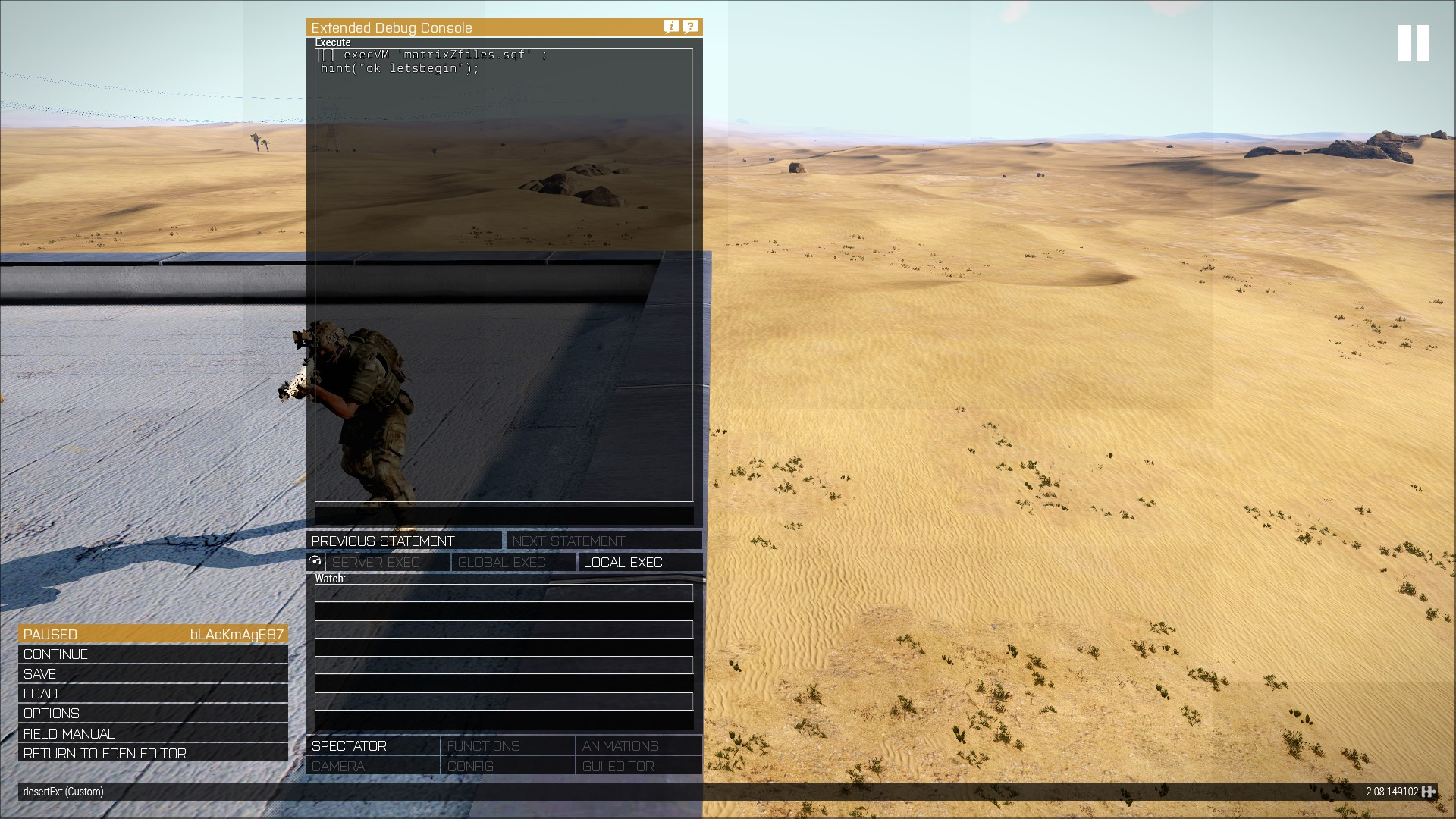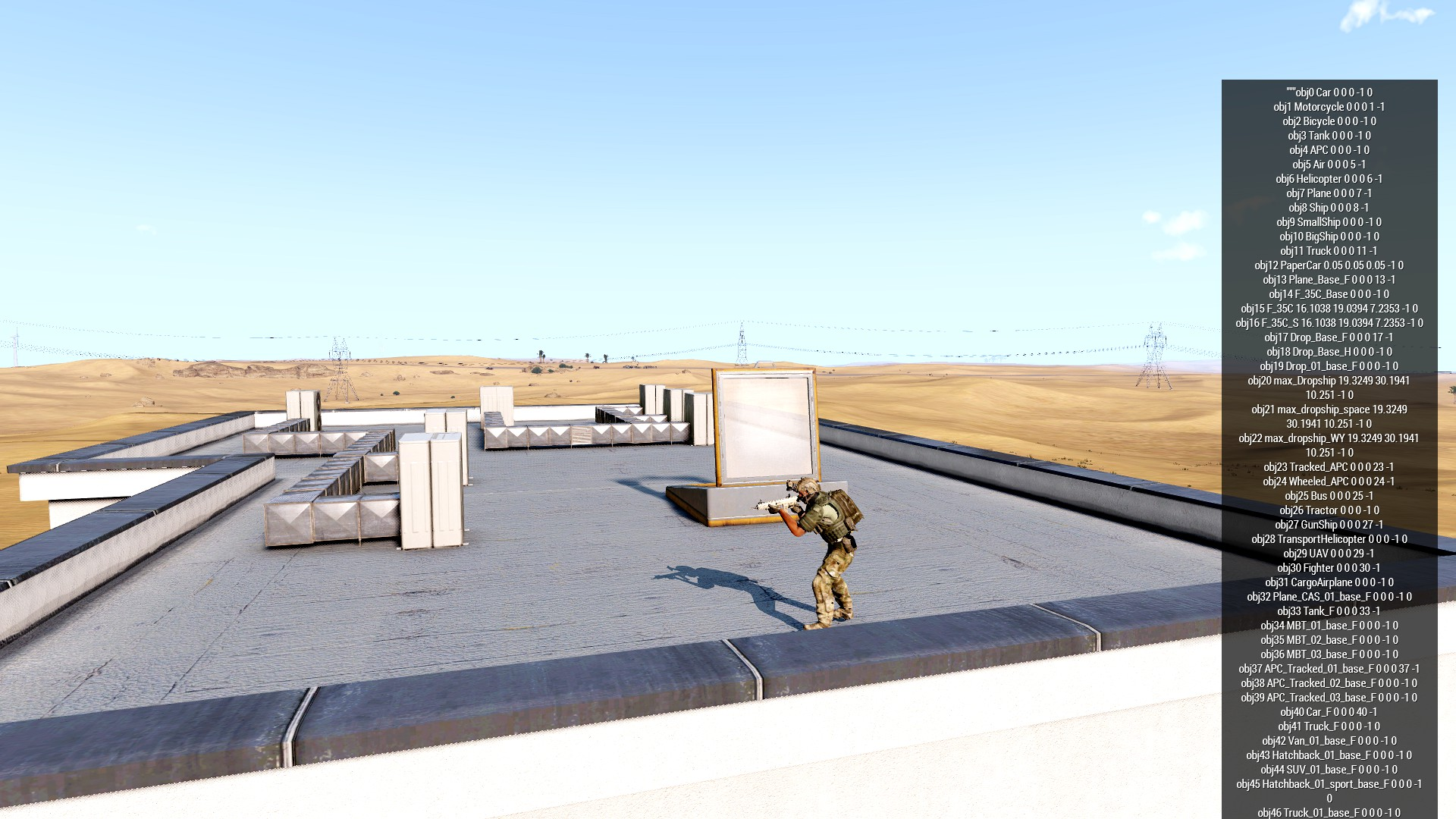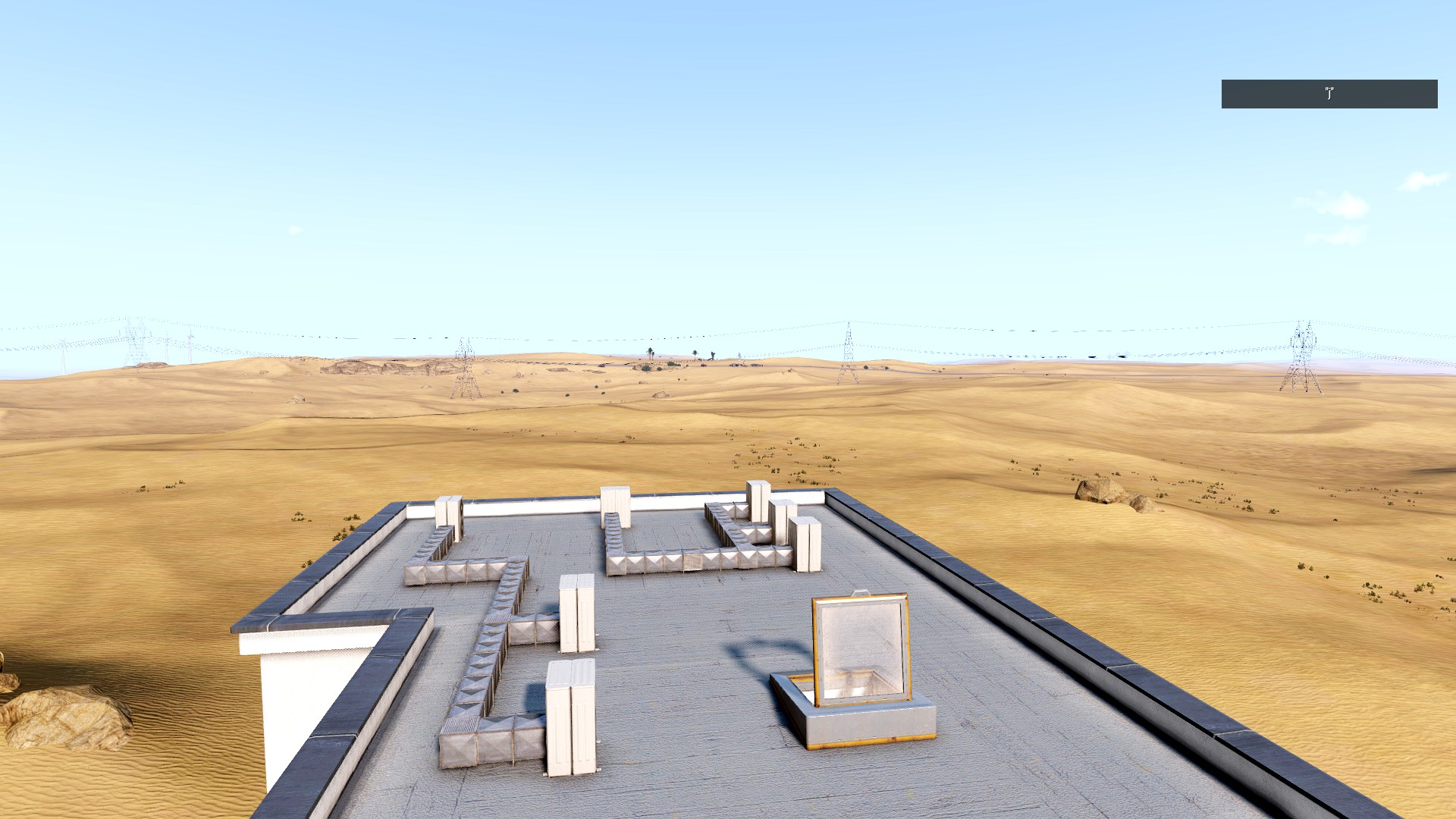CONGRATS BLACKAMAGE! ON THIS DAY YOU EARNED THE RANK OF LANCE CORPORAL!!!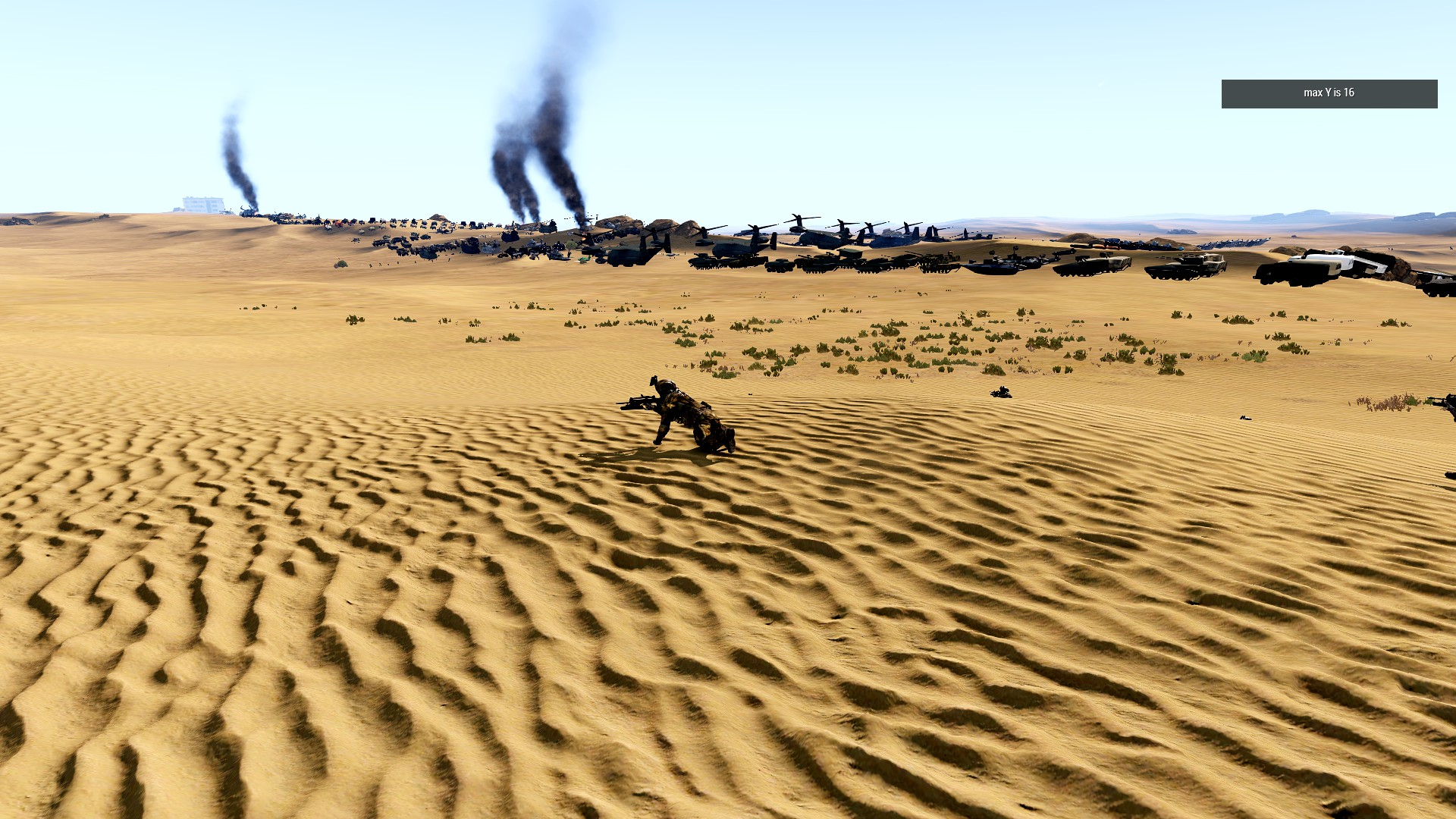##### Share on other sites

Cool concept, this seems like an excellent way to bring in large datasets without adding them directly to scripts. I'll have to play around with this and figure out some good ways to use it

Sidenote: It's really not clear in the post that you are trying to bring forward an interesting command and generate discussion on it. In the past, I have made several tutorial-like posts, you may wish to follow my format or expand upon it and you might have more luck

•1

##### Share on other sites
Posted (edited)

heres the trip up... I got it all cut out... but dunno how to pull the work back out of array... for when I play that final hint... its just a bucket of arrays again...

by the way HERE'S THE TEXT I MADE FROM IN GAME SCRIPT---> USING COPYTOCLIPBOARD

Spoiler
```
<html>
<p>

</p>

</html>```

SAME SCRIPT MORE FUNCTIONALITY

Spoiler
```
private _contents = [] ;

//hint (str _contents) ;

_contentsAry = [] ;
_contentsAry = toArray _contents ;
private _asChars = [] ;
private _asChars = toString _contentsAry ;
_numCharsFile = count _contentsAry ;

private _spotInLine = [] ;

for [{ private  _zx = 0 }, { _zx < _numCharsFile }, { _zx = _zx + 1 }] do
{

private _spotInFile = ""  ;
_spotInFile = ( _contentsAry select _zx ) ;
_spotInLine pushBack _spotInFile ;

//hint (str _spotInFile ) ;

//sleep .03 ;

};

private _asWas = toString _spotInLine ;
//hint (str _asWas ) ;
_numCharsFile2 = count _asWas ;
//sleep 2 ;
hint (str "GO" ) ;
sleep 2 ;

fayz = 0 ; //f
kayz = 0 ; //k
sayx = 0 ; //s

subZ = 0 ; //s
subZf = 0 ; //f-1
private _subwz = "" ;
private _collector =  [] ;
private _spotInFile3 = "" ;
currentSay = 0 ;
for [{ private  _ux = 0 }, { _ux < _numCharsFile2 }, { _ux = _ux + 1 }] do
{

_collecx = [] ;

private _spotBlank = ' '  ;
private _spotInFile2 = ''  ;

waiterUp = _numCharsFile2 - 1 ;
if (_ux !=  waiterUp ) then
{
_spotInFile2 = ( _asWas select [_ux,1] ) ;
_spotInFile3 = ( _asWas select [_ux,2] ) ;
} ;

currentSay = sayx;
if( _spotInFile2 == _spotBlank) then
{
//hint("test" + (str _ux) + (str _spotInFile2)) ;
//blanks duh...
fayz = _ux ;
subZf  = _ux ;
kayz = subZf - (sayx) ;
sayx = fayz + 1  ;
_subwz = _asWas select [ currentSay , kayz] ;
//hint("test" + (str _ux) + (str _subwz)) ;
//sleep (.7) ;
_collecx pushBack _subwz ;

};

if(_spotInFile3 == '\n') then
{
_collector pushBack _collecx ;
sayx = sayx + 2 ;
};

//hint (str _spotInFile2 ) ;

//sleep 2 ;
};

hint("ok end of collect") ;
sleep 4 ;
hint (str _collector) ;

/*
spotAsNum = _spotInFile ;
_spotAsChar = (str _spotInFile );
*/
```

But not getting much faster... so now I'll have to consider simply marking down every spot as a index number in an array. Then pull words out instead of each character. Anything to take away from scanning the array all over again. Putting locks in another file to load seems to be my next path.

Edited by bLAcKmAgE87

##### Share on other sites

Using .txt files to import data is a quite nice thought. I have used it in the past to both save player data (such as steam ID, achievements, qualifications, etc.) as well as bring/read them back in ArmA (as part of a achievement tracking for an ArmA Unit).

Would you care to share the content of the external file to see what is the format before it is imported and compare it to the "end product" (imported array)?

•1

##### Share on other sites

If I  really really wanted to speed things up, I would or could of probably picked a smaller screen, or just change my size down from 32" or what not. The FPS doubles, knowing that, I could find a way or just simply ask how I would start Arma then? I'm know there's way of chunking out codes and making the game run a little more autonomously on single missions. I'm not that good at it. But the point is being that, I should run Arma or some other application in the background, and have it do the paperwork there. Then bring on the game.
The codes explained:

Spoiler

They all came from config>>
In the readout I made I take the dimensions if any using this script ----> (one sec)
A name, number, x y z or zeros are given, a negative one if the next object is  a difference of properties, and the spot where that begins is also appended. All that came from this config file I made ---->>

Spoiler

which I derived from this script--->

Spoiler
```
_gats = [] ;

_gats =  "true" configClasses (configFile >> "CfgVehicles" ) ;
_countEnt = count _gats ;

_dogs = [] ;

_scan = 'bin\config.bin/CfgVehicles/' ;
_countScan = count _scan ;
_type = _scan ;
for [{ _p = 0 }, { _p < _countEnt  }, { _p = _p + 1 }] do
{

_foolu = _gats select _p ;
_strag = (str _foolu) ;
_donk = _strag find _scan  ;
if(_donk != -1) then
{
_dogs pushBack _foolu ;
};
};

_countDogs = count _dogs ;
_passers = []  ;

for [{ _p = 0 }, { _p < _countDogs }, { _p = _p + 1 }] do
{
_stol = _dogs select _p ;
_stool = (str _stol) ;

_newBoyC = count _stool ;
_newBoyC = _newBoyC - _countScan ;
_newBoyC = _newBoyC  ;

_donker = _stool select [ _countScan , _newBoyC ] ;

_passers pushBack _donker ;

};

_wasted = [] ;
_wasted2 = [] ;
_wasted3 = [] ;
for [{ _g = 0 }, { _g < _countDogs }, { _g = _g + 1 }] do
{
sloty = _passers select _g ;
_configs = configProperties [configFile >> "CfgVehicles" >> sloty ];
_configs2 =  getNumber (configFile >> "CfgVehicles" >> sloty >> "fuelCapacity" ) ;
_configs3 = getText (configFile >> "CfgVehicles" >> sloty >> "_generalMacro" ) ;
_wasted pushBack _configs ;
_wasted2 pushBack _configs2 ;
_wasted3 pushBack _configs3 ;
};

_spotty = _wasted select 0 ;

_fuul = [] ;

for [{ _v = 0 }, { _v < _countDogs }, { _v = _v + 1 }] do
{
_slotzz = _passers select _v ;
_slotzv = _wasted2 select _v ;

_scanz = '1' ;
_spoz = (str _slotzv );
_donkz = _spoz find _scanz ;

if( _slotzv > 0) then
{
_fuul pushBack _slotzz ;

};

};

copyToClipboard (str _fuul ) ; ﻿

_countTankX = co﻿unt ( _fuul ) ;
_treesW = 0 ;
_treesL = 0 ;
_rackX = _treesW ;
_rackY =  _treesL ;

_racks = _rackX * _rackY ;
_bumper = 1 ;

_markST = getPos towerSt;

whax =   0 ;
whay =   0 ;
whaz =   0 ;

rackBumpX = 2 ;
rackBumpY = 2 ;

whax = ( _markST select 0 ) ;
whay = ( _markST select 1 )  ;
whay = whay - 100 ;

halfX = rackBumpX / 2 ;
halfY = rackBumpY / 2 ;
whaLoc = whax ;
whaLocY = whay ;
halfDog =  2 ;

_blame = "" ;
_blame2 = "" ;
_blameX = "" ;
_blameX2 = "" ;

_longs = [] ;

_positionRemainY = [(whax),(whay),(whaz)] ;
_positionRemainX = [(whax),(whay),(whaz)] ;
_positionsXY = [] ;

lastW = 0 ;
lastL = 0 ;
lastWx = 0 ;
lastLx = 0 ;
rowPing = 0 ;

hint("loop layer starting") ;

_dimenzOf = [] ;
_dimenzRow = [] ;

bumperSpot = 0 ;

_stringTesting = "" ;

for [{private _gh = 0 }, { _gh < _countTankX }, { _gh = _gh + 1 }] do
{
bumperSpot = -1 ;

_shells = 0 ;
_moments = []  ;
slotG = _fuul select _gh ;
_inPar = _fuul select _gh ;
_fun = (str _inPar) ;

_configGM = getText (configFile >> "CfgVehicles" >> slotG >> "editorSubcategory" ) ;
_configXM = getText (configFile >> "CfgVehicles" >> slotG >> "_generalMacro" ) ;
_blame = _configGM ;
_blamer = _blame2  ;
_blame2 = _blame ;
_blameX = _configXM ;
_blameXr = _blameX2  ;
_blameX2 = _blameX ;
_welp = _blame find _blamer ;
if(_welp < 0 ) then
{
_gelp = _blameX find _blameXr ;
if( _gelp < 0  ) then
{
if(_gh  != 0) then
{
bumperSpot = _gh ;

_shells = _gelp ;
_dimenzRow pushBack _gh ;
};
};
} ;

private _posNews = [(0),(0),(0)] ;
private _blamz = "obj"+ (str _gh)  ;
private _blamor = _blamz;

_blamz = createVehicle [_inPar,_posNews ,[],0,"CAN_COLLIDE"];
private _dimenz = boundingBoxReal _blamz ;

//https://community.bistudio.com/wiki/sizeOf
//vs. using boundingBox or BoundingBoxReal
// real which causes a lot of zeros to be read off as size

_blamz enableSimulation false ;
_blamz allowDamage false ;
_blamz hideObject true ;

private _z1 = _dimenz select 0;
private _z2 = _dimenz select 1;

private _zW = abs ((_z2 select 0) - (_z1 select 0));
private _zL = abs ((_z2 select 1) - (_z1 select 1));
private _zH = abs ((_z2 select 2) - (_z1 select 2));

_moments pushBack _blamor ;
_moments pushBack _inPar ;
_moments pushBack _zW ;
_moments pushBack _zL ;
_moments pushBack _zH ;
_moments pushBack bumperSpot ;
_moments pushBack _shells ;

_dimenzOf pushBack _moments ;
_stringTesting = _stringTesting + _blamor + " " + _inPar  + " " + (str _zW) + " " + (str _zL) + " " + (str _zH) + " " + (str bumperSpot) + " " + (str _shells) + " \n" ;
//deleteVehicle _blamz ;

};

hint (str _stringTesting) ;
copyToClipBoard (str _stringTesting) ; ```

...which is actually designed to create both arrays at once. Notice that it uses another array, that is, it marks on another array: Just the detected differences. So that the numbers are logically aligned to use this script---->

Here is the main loading script re-done... not quite done yet, but a few minutes faster...
Takes only 45s in comparison to 4 mins.

Spoiler
```
private _contents = [] ;

//hint (str _contents) ;

_contentsAry = [] ;
_contentsAry = toArray _contents ;
_numCharsFile = count _contentsAry ;

private _asWas = toString _contentsAry ;

_numCharsFile2 = count _asWas ;
hint (str "GO" ) ;

sleep 2 ;

fayz = 0 ; //f
kayz = 0 ; //k
sayx = 0 ; //s

subZ = 0 ; //s
subZf = 0 ; //f-1
private _subwz = "" ;

waiterUp = _numCharsFile2 - 1 ;
private _spotInFile1 = "" ;
private _spotInFile2 = "" ;
currentSay = 0 ;
private _collector =  [] ;
private _collecx = [] ;

for [{ private  _ux = 0 }, { _ux < _numCharsFile2 }, { _ux = _ux + 1 }] do
{
if (_ux !=  waiterUp ) then
{

private _spotBlank = ' '  ;

_spotInFile1 = ( _asWas select [_ux,1] ) ;
_spotInFile2 = ( _asWas select [_ux,2] ) ;

currentSay = sayx;
if( _spotInFile1 == _spotBlank) then
{

fayz = _ux ;
subZf  = _ux ;
kayz = subZf - (sayx) ;
sayx = fayz + 1  ;
_subwz = _asWas select [ currentSay , kayz] ;

_collecx pushBack _subwz ;

};

if(_spotInFile2 == '\n') then
{	_fail = [] ;
_ohMan = "" ;
_ohMan2 = "" ;
{
_ohMan = [_x] ;
_fail pushBack _ohMan ;

} forEach _collecx ;

//hint("test" + (str _ux) + (str _fail)) ;

_collector pushBack _fail ;

_collsize = (count _collecx) ;
_collecx deleteRange[0, _collsize] ;
sayx = sayx + 2 ;
};

} ;
};

hint("ok end of collect") ;
sleep 4 ;
hint (str _collector) ;

/*
But not getting much faster... so now I'll have to consider simply marking down every spot as a index number in an array. Then pull words out instead of each character. Anything to take away from scanning the array all over again. Putting locks in another file to load seems to be my next path.

*/```

... So from here I got what I want... but it could be just a bit more.... preloaded... I'll write another part now... where all the row changes get an array like the other boneyard script. Then I'll just write out the indices in an another array again.
The script should go through the number of row counts in a FOR loop.
Pulls out the the numbers from the array... the character positions again.

Select the whole copied file from the those two points and append a string to an array.

But my idea is flawed if I want faster.... because then I'll have an array of single strings and no nests.
The problem of breaking each string into individual values quickly rolls back along.

Is this quicker than just adding arrays like I am? Probably not... but I could always format the text some more
and write a structured text... then copy that into a text file.. then create the array automatically from there using  instantiation.
(no much in the way of default constructors but hey! )

One tool that I should consider using-->  https://community.bistudio.com/wiki/createHashMapFromArray
Another one I should be using --> https://community.bistudio.com/wiki/arrayIntersect
As well as --> https://community.bistudio.com/wiki/BIS_fnc_subSelect

Anything that takes an array and breaks it apart into another array.

I think that preloading arrays is the fastest way, so there's always
the time it takes the first time around, or to refresh your grid.

Here is the array getting automatically created.... but i noticed a little mistake in the very start... see it?

Spoiler

And here is the simple loader

Spoiler
```
private _contentZ = [] ;
_failZ = toArray _contentZ ;
hint (_contentZ) ;```

##### Share on other sites

Hey, that's a lot of information and I am not sure I completely follow your approach or what exactly you are trying to achieve.

I do see some redundancy in the first script you provided in the last answer (for example you have a lot of for-loops that iterate through the same array and you don't really use data from the previous iterations, which means you could possibly fit everything in a single go of the for-loop. Additionally you could use findIf instead of find which is a wee bit faster, especially for large arrays) but I may be mistaken because I don't get exactly what you are trying to achieve.

Thanks for sharing though.

•2

##### Share on other sites
Posted (edited)
19 hours ago, ZaellixA said:

Hey, that's a lot of information and I am not sure I completely follow your approach or what exactly you are trying to achieve.

I do see some redundancy in the first script you provided in the last answer (for example you have a lot of for-loops that iterate through the same array and you don't really use data from the previous iterations, which means you could possibly fit everything in a single go of the for-loop. Additionally you could use findIf instead of find which is a wee bit faster, especially for large arrays) but I may be mistaken because I don't get exactly what you are trying to achieve.

Thanks for sharing though.

Yea it goes through a lot of redundant code
... all I'm doing is taking a text I made from a script, and then turning it into a useable array with all the config>>vehicle names.
Then I can spawn them.
Not only that. They are sorted by not only their names, but by their properties such as "hasFuel" or "canCarryBackpark"... don't get me wrong.. I couldn't really shorten it up much without errors being thrown. It looks like the same array  but they started off as self-constructing and always create another array or modify another.

Yea, in reality I could take all those for loops and make them a lot more modular, but for demonstration purposes I have them out in blocks.

This whole thing doesn't work out btw. I"m still working it. It would be nice to use pointers .

Here is where am I at...

Spoiler
```
private _contentZ = [] ;
_failZ = toArray _contentZ ;

private _rowZ = [] ;
_failRZ = toArray _rowZ ;

_dimenzOf = [] ;
_dimenzRow = [] ;

_dimenzOf = toString _failZ ;
_dimenzRow = toString _failRZ ;

_countTankX = count ( _failZ ) ;

_stringTesting = "" ;

_treesW = 0 ;
_treesL = 0 ;
_rackX = _treesW ;
_rackY =  _treesL ;

_racks = _rackX * _rackY ;
_bumper = 1 ;

_markST = getPos towerSt;

whax =   0 ;
whay =   0 ;
whaz =   0 ;

/*
_inParts = _fuul select _po;
_funs = (str _inParts ) ;
*/

rackBumpX = 2 ;
rackBumpY = 2 ;

whax = ( _markST select 0 ) ;
whay = ( _markST select 1 )  ;
whay = whay - 100 ;

halfX = rackBumpX / 2 ;
halfY = rackBumpY / 2 ;
whaLoc = whax ;
whaLocY = whay ;
halfDog =  2 ;

_longs = [] ;

_positionRemainY = [(whax),(whay),(whaz)] ;
_positionRemainX = [(whax),(whay),(whaz)] ;
_positionsXY = [] ;

lastW = 0 ;
lastL = 0 ;
lastWx = 0 ;
lastLx = 0 ;
rowPing = 0 ;

hint("loop layer starting") ;

bumperSpot = 0 ;

_lessThanCount = _countTankX - 1 ;
startMark = 0 ;
rememberMark = 0 ;

hint ( str(whax) + "\n" + str(whay) ) ;

sleep 2 ;

whacx = 0 ;
whacy = 0 ;
knox  = 0;
knoy = 0;
knoz = 0;

hint (str _dimenzOf ) ;
sleep 2 ;
//copyToClipboard(_dimenzOf) ;

hint (str _dimenzRow ) ;
sleep 2 ;

//_funny = _dimenzOf select 16 ;
//hint (str _funny) ;
//sleep 2 ;

for [{private _po = 0 }, { _po < _countTankX }, { _po= _po+ 1 }] do
{

hint ( str(whax) + "123\n" + str(whay) ) ;
//sleep 5 ;
whacx = whax + 0  ;
hint("wait...block") ;
//sleep 2 ;
whacy = (whay) + 0 ;
hint("wait...block 2") ;
//sleep 5 ;
_objNumbez = "" ;
_objNamez = "" ;
tw = 0 ;
tl = 0 ;
th = 0 ;
private _objNext = 0 ;
private _rowIsNext = 0 ;

_party = _dimenzOf select _po;

_objNumbez = _party select 0  ;
_objNamez = _party select 1 ; //literal strings up to here
tw = _party select 2 ; //all strings pass here from numbers
tl = _party select 3 ;
th = _party select 4 ;
_objNext = _party select 5 ;
//_rowIsNext = _party select 6 ;

private _posNex = [(whacx),(whacy),(whaz)] ;
private _funGuy = _objNumbez + "x" ;
hint (str(_funGuy)) ;
//sleep 4 ;

_bop = _po;
_bopo = _lessThanCount ;

if ( _bop != _bopo ) then
{

_fu = (_bop) + 1 ;
_goers = ( _dimenzOf select _fu) ;

_rowIsNext = _goers select 6 ;
_objNext = _goers select 5 ;
hint(str _goers) ;
//sleep 4 ;

};

_gonerz = [] ;
_gonerz = _dimenzOf select _po ;
_gz = _gonerz select 3 ;
if( _rowIsNext < 0 && _bop != _bopo) then
{

startMark = rememberMark ;
rememberMark = _po;

hint("row is next...\n" + (str startmark) + " as start mark\n" + (str rememberMark) + " as r mark...") ;
//sleep 3 ;
//alternatively i could just add the max width to the array for the end... but o well
_lidx = [] ;
_lidx2 = [] ;

private _nextRowStart = 0 ;
private _nextRowStart = _po  + 1 ;
private _nextRowEnd = 0 ;

private _yougotit = _dimenzRow find _nextRowStart  ;
hint ("_yougotit = " + (str _yougotit)) ;
//sleep 2;
if(  _yougotit > 0 ) then
{
if( _po != 0) then
{

nextGet = 0 ;
nextGet = _yougotit + 1 ;
nexter = _dimenzRow select nextGet ;
_nextRowEnd = nexter ;
hint ("_nextRowEnd  = " + (str _nextRowEnd ) + "_nextRowStart  = " + (str _nextRowStart )) ;
//sleep 2;
};
};

private _combx = 0 ;
_combx =  _nextRowEnd - (_nextRowStart) ;
hint ("_combx = " + (str _combx )) ;
//sleep 2;
private _maxLidx2 =  0 ;
if ( _combx > 0 ) then
{
hint("combx loop") ;
//sleep 2 ;
for [{private _gq = _nextRowStart }, { _gq <= _nextRowEnd }, { _gq = _gq + 1 }] do
{
_gonet = [] ;
_gonet = _dimenzOf select _gq ;

_gb = _gonet select 3 ;
_lidx2  pushBack _gb ;

};

_maxLidx2 =  selectMax _lidx2 ;
};

for [{private _gf = startMark  }, { _gf <= rememberMark }, { _gf = _gf + 1 }] do
{
_goners = [] ;
_goners = _dimenzOf select _gf ;

_gl = _goners select 3 ;
_lidx  pushBack _gl ;

};

private _maxLidx = selectMax _lidx ;

hint(str( _lidx  ));
//sleep 3;

//_maxLidx2 = 0 ;
hint("max Y is " + (str _maxLidx) ) ;
//sleep 3;
if ( _gz != 0) then
{

_maxLidx = _maxLidx / 2 ;
_maxLidx2 = _maxLidx2 / 2 ;
mucky = rackBumpY + _maxLidx  ;
mucky = mucky + (_maxLidx2) ;
whay = whay - mucky ;
whax = whaLoc ;

};

};

_inParts = _objNamez ;

private _blarz = (str _po ) + "obj";
if ( _gz != 0) then
{
private _nextWid = 0 ;
_blarz = createVehicle [ _inParts , _posNex ,[],0,"CAN_COLLIDE"];
_blarz enableSimulation false ;
spaceW = tw ;
spaceW = spaceW ;
if( _rowIsNext > 0) then
{
bumpPo = _po + 1 ;
_gon = [] ;
_gon = _dimenzOf select bumpPo  ;

_goo = _goo select 2 ;
_nextWid = _goo ;
_nextWid = _nextWid  ;
};
spaceW = spaceW + rackBumpX + _nextWid  ;

whax =   whax + spaceW ;

};

//_positionsXY pushBack _posNex ;

};

hint("done with it") ;```

This will take the text files and turn them into arrays. Instead of creating a bunch of arrays with data to use they are there already and no new objects need be made.
Actually, once text gets to the array, I pull data from another array and retrieve dimensions. The code
scans ahead and gets the next object's dimensions, then it puts objects down so that they don't run into each ever.
For rows, the same thing almost, pull the data out. For a objects iteration, a row detect is given, if true then the code will

scan ahead again and get all the lengths of the next row (as well as the one your on). Then the row
distance is given and the next object CAN be created back in the next ORIGINAL coloumn spot ...............(whaLoc)

So many things at once! I'll need be more discrete.

English is a programming language.

Edited by bLAcKmAgE87
5/24/22 0210

##### Share on other sites
Posted (edited)

https://community.bistudio.com/wiki/parseSimpleArray
ok getting hungry

This is the working code. Loads all the vehicles sorted out in rows in 30 seconds in or less about.  Uses the file I made from another script. I'll post the exact script which makes the text file later soon. I'll also clean up the code as I go along. Read if you wish.

I use an object called towerSt from the editor and start my mish running .... arrayMatrix.sqf .... that's what I called it in this version.
The deficit: You can go through my older posts and see that it becomes a trigonometric challenge when ordering arrays of objects on the map.

They face the same direction everytime and so does the entire array. I'll fiix this up once I clean up this code. That... is all. Orientation leads to more math and calculus.

Also ...to test it fast... I gave a max count of like a 30 configs.... the errors that came up got me in the end tho..
I still gotta keep it from bumping ahead when there are no more rows ahead... something that hasn't quite worked right....

This one just helps me create an array to cut and paste... I still can't write any texts with Arma... perhaps someone knows a  XML code to make that work.

Spoiler
```
_gats = [] ;

_gats =  "true" configClasses (configFile >> "CfgVehicles" ) ;
_countEnt = count _gats ;

_dogs = [] ;

_scan = 'bin\config.bin/CfgVehicles/' ;
_countScan = count _scan ;
_type = _scan ;
for [{ _p = 0 }, { _p < _countEnt  }, { _p = _p + 1 }] do
{

_foolu = _gats select _p ;
_strag = (str _foolu) ;
_donk = _strag find _scan  ;
if(_donk != -1) then
{
_dogs pushBack _foolu ;
};
};

_countDogs = count _dogs ;
_passers = []  ;

for [{ _p = 0 }, { _p < _countDogs }, { _p = _p + 1 }] do
{
_stol = _dogs select _p ;
_stool = (str _stol) ;

_newBoyC = count _stool ;
_newBoyC = _newBoyC - _countScan ;
_newBoyC = _newBoyC  ;

_donker = _stool select [ _countScan , _newBoyC ] ;

_passers pushBack _donker ;

};

//_countDogs =100 ;
_wasted = [] ;
_wasted2 = [] ;
_wasted3 = [] ;
for [{ _g = 0 }, { _g < _countDogs }, { _g = _g + 1 }] do
{
sloty = _passers select _g ;
_configs = configProperties [configFile >> "CfgVehicles" >> sloty ];
_configs2 =  getNumber (configFile >> "CfgVehicles" >> sloty >> "fuelCapacity" ) ;
_configs3 = getText (configFile >> "CfgVehicles" >> sloty >> "_generalMacro" ) ;
_wasted pushBack _configs ;
_wasted2 pushBack _configs2 ;
_wasted3 pushBack _configs3 ;
};

_spotty = _wasted select 0 ;

_fuul = [] ;

for [{ _v = 0 }, { _v < _countDogs }, { _v = _v + 1 }] do
{
_slotzz = _passers select _v ;
_slotzv = _wasted2 select _v ;

_scanz = '1' ;
_spoz = (str _slotzv );
_donkz = _spoz find _scanz ;

if( _slotzv > 0) then
{
_fuul pushBack _slotzz ;

};

};

_countTankX = count ( _fuul ) ;

_markST = getPos towerSt;

_blame = "" ;
_blame2 = "" ;
_blameX = "" ;
_blameX2 = "" ;

lastW = 0 ;
lastL = 0 ;
lastWx = 0 ;
lastLx = 0 ;
rowPing = 0 ;

hint("loop layer starting") ;

_dimenzOf = [] ;
_dimenzRow = [] ;

bumperSpot = 0 ;

private _objNamePass = "" ;

for [{private _gh = 0 }, { _gh < _countTankX }, { _gh = _gh + 1 }] do
{
bumperSpot = -1 ;

_shells = 0 ;
_moments = []  ;
slotG = _fuul select _gh ;
_inPar = _fuul select _gh ;
_fun = (str _inPar) ;

_configGM = getText (configFile >> "CfgVehicles" >> slotG >> "editorSubcategory" ) ;
_configXM = getText (configFile >> "CfgVehicles" >> slotG >> "_generalMacro" ) ;
_blame = _configGM ;
_blamer = _blame2  ;
_blame2 = _blame ;
_blameX = _configXM ;
_blameXr = _blameX2  ;
_blameX2 = _blameX ;
_welp = _blame find _blamer ;
if(_welp < 0 ) then
{
_gelp = _blameX find _blameXr ;
if( _gelp < 0  ) then
{
if(_gh  != 0) then
{
bumperSpot = _gh ;

_shells = _gelp ;
_dimenzRow pushBack _gh ;
};
};
} ;

private _posNews = [(0),(0),(0)] ;
_objNamePass = 'xzobj'+ (str _gh)  ;
private _blamz = 'zobj'+ (str _gh)  ;
private _blamor = _objNamePass   ;

_blamz = createVehicle [_inPar,_posNews ,[],0,"CAN_COLLIDE"];
private _dimenz = boundingBoxReal _blamz ;

_blamz enableSimulation false ;
_blamz allowDamage false ;
_blamz hideObject true ;

private _z1 = _dimenz select 0;
private _z2 = _dimenz select 1;

private _zW = abs ((_z2 select 0) - (_z1 select 0));
private _zL = abs ((_z2 select 1) - (_z1 select 1));
private _zH = abs ((_z2 select 2) - (_z1 select 2));

_mb = [] ;
_mi = [] ;
_mw = [] ;
_ml = [] ;
_mh = [] ;
_mbs = [] ;
_msh = [] ;

_mb pushBack (  _blamor) ;
_mi pushBack ( _inPar );
_mw pushBack (str _zW );
_ml pushBack (str _zL );
_mh pushBack (str _zH );
_mbs pushBack (str bumperSpot) ;
_msh pushBack (str _shells );

_moments pushBack ( _mb ) ;
_moments pushBack ( _mi );
_moments pushBack ( _mw );
_moments pushBack ( _ml );
_moments pushBack ( _mh );
_moments pushBack ( _mbs ) ;
_moments pushBack ( _msh );

_dimenzOf pushBack ( _moments) ;

//deleteVehicle _blamz ;

};

hint (str _fuul ) ;
copyToClipBoard (str _fuul ) ;
sleep 2 ;

hint (str _dimenzOf ) ;
copyToClipBoard (str _dimenzOf ) ;
sleep 6 ;

//_dimenzOf = toString (toArray _dimenzOf) ;
//hint(str _dimenzOf) ;

hint (str _dimenzRow ) ;
copyToClipBoard (str _dimenzRow ) ;
sleep 6 ;

/*

[[{"xzobj0"},{"Car"},{0},{0},{0},{-1},{0}],[{"xzobj1"},{"Motorcycle"},{0},{0},{0},{1},{-1}],[{"xzobj2"},{"Bicycle"},{0},{0},{0},{-1},{0}],[{"xzobj3"},{"Tank"},{0},{0},{0},{-1},{0}],[{"xzobj4"},{"APC"},{0},{0},{0},{-1},{0}],[{"xzobj5"},{"Air"},{0},{0},{0},{5},{-1}],[{"xzobj6"},{"Helicopter"},{0},{0},{0},{6},{-1}],[{"xzobj7"},{"Plane"},{0},{0},{0},{7},{-1}],[{"xzobj8"},{"Ship"},{0},{0},{0},{8},{-1}],[{"xzobj9"},{"SmallShip"},{0},{0},{0},{-1},{0}],[{"xzobj10"},{"BigShip"},{0},{0},{0},{-1},{0}],[{"xzobj11"},{"Truck"},{0},{0},{0},{11},{-1}],[{"xzobj12"},{"PaperCar"},{0.05},{0.05},{0.05},{-1},{0}],[{"xzobj13"},{"Plane_Base_F"},{0},{0},{0},{13},{-1}],[{"xzobj14"},{"F_35C_Base"},{0},{0},{0},{-1},{0}],[{"xzobj15"},{"F_35C"},{13.4198},{15.8662},{6.02942},{-1},{0}],[{"xzobj16"},{"F_35C_S"},{13.4198},{15.8662},{6.02942},{-1},{0}]]

*/
```

Here is the strip from config.... nothing in terms of order other than the next mod.  If you don't want such a huge text file copy then just the first 100 instead of every single config. Otherwise... this script should take about 5 mins..
That is why I wrote the next script.

Spoiler

Here is the array that uses data from the config strip.. marks are as follows :[ IndexPreName, ObjName, xsize, y, z, nextItemChanges, ifPrevChangedThisRowStartsIndex ]

Spoiler

Here is the array in a text marking a index for every spot where properties and identities change enough to bump rows. Did I say rows? Why not columns?( ; ( )

Spoiler
Spoiler

[1,5,6,7,8,11,13,17,23,24,25,27,29,30,33,37,40,58,62,66,67,70,71,74,75,78,79,85,138,143,146,147,149,150,152,159,161,163,178,182,189,292,294,334,346,354,361,369,372,376,400,407,408,419,444,449,557,561,564,565,568,572,578,607,608,611,612,615,617,620,621,638,640,642,660,663,666,669,672,678,680,682,688,690,696,700,702,703,705,706,707,710,718,720,728,731,733,739,740,760,766,767,782,784,790,792,793,800,825,826,841,849,850,859,860,861,862,863,864,865,866,868,870,880,882,886,888,889,898,904,968,969,977,978,979,982,984,987,989]

Finally the code you can get used to using once you have your hard data down.. this script will create a field of objects using all that data in such a way that nothing collides with itself or is disorganized.
Still lacks a lot of detail and results.... but it works.

Spoiler
```
private _contentZ = [] ;
_contentsAry = [] ;
_contentsAry = toArray _contentZ ;
private _asWas = toString _contentsAry ;
_contentZZ = parseSimpleArray  _asWas ;

private _contentRZ = [] ;
_contentsRow = [] ;
_contentsRow = toArray _contentRZ ;
private _rasWas = toString _contentsRow ;
_contentRR = parseSimpleArray  _rasWas ;

//hint ( str _contentZZ ) ;
//sleep 1;

private _dimenzOf = [] ;
private _dimenzRow = [] ;

_dimenzOf = _contentZZ  ;
_dimenzRow = _contentRR  ;

_countTankX = count ( _dimenzOf ) ;

_stringTesting = "" ;

_treesW = 0 ;
_treesL = 0 ;
_rackX = _treesW ;
_rackY =  _treesL ;

_racks = _rackX * _rackY ;
_bumper = 1 ;

_markST = getPos towerSt;

whax =   0 ;
whay =   0 ;
whaz =   0 ;

/*
_inParts = _fuul select _po;
_funs = (str _inParts ) ;
*/

rackBumpX = 2 ;
rackBumpY = 2 ;

whax = ( _markST select 0 ) ;
whay = ( _markST select 1 )  ;
whay = whay - 100 ;

halfX = rackBumpX / 2 ;
halfY = rackBumpY / 2 ;
whaLoc = whax ;
whaLocY = whay ;
halfDog =  2 ;

_longs = [] ;

_positionRemainY = [(whax),(whay),(whaz)] ;
_positionRemainX = [(whax),(whay),(whaz)] ;
_positionsXY = [] ;

lastW = 0 ;
lastL = 0 ;
lastWx = 0 ;
lastLx = 0 ;
rowPing = 0 ;

hint("loop layer starting") ;
sleep (1.5) ;
bumperSpot = 0 ;

_lessThanCount = _countTankX - 1 ;
startMark = 0 ;
rememberMark = 0 ;

hint ( str(whax) + "\n" + str(whay) ) ;

sleep (1.5) ;

whacx = 0 ;
whacy = 0 ;
knox  = 0;
knoy = 0;
knoz = 0;

//hint (str _dimenzOf) ;

//copyToClipboard (str _dimenzOf) ;

//sleep (1.5) ;

//hint (str _dimenzRow ) ;

//sleep (1) ;

hint (str _countTankX + "...counter") ;
sleep 2;

for [{private _po = 0 }, { _po < _countTankX }, { _po= _po+ 1 }] do
{

//hint ( str(whax) + "123\n" + str(whay) ) ;
//sleep 1 ;
whacx = whax + 0  ;
//hint("wait...block") ;
//sleep (.4) ;
whacy = (whay) + 0 ;
//hint("wait...block 2") ;
//sleep 5 ;
_objNumbez = "" ;
_objNamez = "" ;
tw = 0 ;
tl = 0 ;
th = 0 ;
_objNext = 0 ;
_rowIsNext = 0 ;

_party = _dimenzOf select _po;

_objNumbez = ( _party select 0 ) select 0  ;
hint (str _objNumbez) ;
//sleep (.4) ;

_objNamez = (_party select 1 ) select 0 ; //literal strings up to here
//hint (str _objNamez ) ;
//sleep (.4) ;

tw =  (_party select 2 ) select 0 ; //all strings pass here from numbers
tl =  (_party select 3 ) select 0 ;
th = (_party select 4 ) select 0 ;
_objNext = ( _party select 5) select 0 ;
//_rowIsNext = _party select 6 ;

tw =parseNumber  tw ;
tl =parseNumber  tl ;
th =parseNumber  th ;
_objNext = parseNumber _objNext  ;

hint("w= " + str tw + "\n" + "l= "  + str tl + "\n" + "h= " + str th ) ;
//sleep (.4) ;

hint("obj next= " + str _objNext) ;
//sleep (.4) ;

private _posNex = [(whacx),(whacy),(whaz)] ;
private _funGuy = (_objNumbez) + "x" ;

//_soot = compile _funGuy ;

_bop = _po;
_bopo = _lessThanCount ;

if ( _bop != _bopo ) then
{

_fu = (_bop) + 1 ;

_goers = ( _dimenzOf select _fu) ;

_rowIsNext =  ( _goers select 6 ) select 0;
_rowIsNext = parseNumber _rowIsNext ;
_objNext =  (_goers select 5 ) select 0;
_objNext = parseNumber _objNext  ;
//hint(str _goers) ;

//sleep 4 ;

};

_gonerz = [] ;
_gonerz = _dimenzOf select _po ;
_gz = (_gonerz select 3 ) select 0 ;
_gz = parseNumber _gz ;
//hint (str _gz) ;
//sleep 2 ;

if( _rowIsNext < 0 && _bop != _bopo) then
{

startMark = rememberMark ;
rememberMark = _po;

//hint("row is next...\n" + (str startmark) + " as start mark\n" + (str rememberMark) + " as r mark...") ;
//sleep 3 ;
//alternatively i could just add the max width to the array for the end... but o well
_lidx = [] ;
_lidx2 = [] ;

private _nextRowStart = 0 ;
private _nextRowStart = _po  + 1 ;
private _nextRowEnd = 0 ;

private _yougotit = _dimenzRow find ( _nextRowStart ) ;
//hint ("_yougotit = " + (str _yougotit)) ;
//sleep (.05);
if(  _yougotit > 0 ) then
{
if( _po != 0  ) then
{
_countTankXX = _countTankX - 2 ;
if(_po < _countTankXX ) then
{
try
{
nextGet = 0 ;
nextGet = _yougotit + 1 ;
nexter = _dimenzRow select nextGet ;
nexter = nexter ;
_nextRowEnd = nexter ;
} catch
{
_nextRowEnd = 1 ;

};

//hint ("_nextRowEnd  = " + (str _nextRowEnd ) + "_nextRowStart  = " + (str _nextRowStart )) ;
//sleep (.05);
};

//???wtd
if(_po == _countTankXX ) then
{
_nextRowEnd = 2 ;
_nextRowStart = 0 ;
};

};
};

private _combx = 0 ;
private _maxLidx2 =  0 ;
try {
_combx =  (_nextRowEnd ) - (_nextRowStart) ;
if ( _combx > 0 ) then
{
hint("combx loop") ;
//sleep (.05) ;
for [{private _gq = _nextRowStart }, { _gq <= _nextRowEnd }, { _gq = _gq + 1 }] do
{
_gonet = [] ;
_gonet =  (_dimenzOf select _gq) ;

_gb =  ( _gonet select 3) select 0 ;
_gb = parseNumber _gb ;
_lidx2  pushBack _gb ;

};

_maxLidx2 =  selectMax _lidx2 ;
};
}
catch {
_combx = 0 ;
};

//hint ("_combx = " + (str _combx )) ;
//sleep (2);

for [{private _gf = startMark  }, { _gf <= rememberMark }, { _gf = _gf + 1 }] do
{
_goners = [] ;
_goners = _dimenzOf select _gf ;

_gl = (_goners select 3 ) select 0 ;
_gl = parseNumber _gl ;
_lidx  pushBack _gl ;

};

private _maxLidx = selectMax _lidx ;

//hint(str( _lidx  ));
//sleep 3;

//_maxLidx2 = 0 ;
//hint("max Y is " + (str _maxLidx) ) ;
//sleep 3;
if ( _gz != 0) then
{

_maxLidx = _maxLidx / 2 ;
_maxLidx2 = _maxLidx2 / 2 ;
mucky = rackBumpY + _maxLidx  ;
mucky = mucky + (_maxLidx2) ;
whay = whay - mucky ;
whax = whaLoc ;

};

};

_inParts = _objNamez ;

private _blarz = (str _po ) + "obj";
if ( _gz != 0) then
{
private _nextWid = 0 ;
_blarz = createVehicle [ _inParts , _posNex ,[],0,"CAN_COLLIDE"];
_blarz enableSimulation false ;
spaceW = tw ;
spaceW = spaceW ;
if( _rowIsNext > 0) then
{
bumpPo = _po + 1 ;
_gon = [] ;
_gon = _dimenzOf select bumpPo  ;

_goo =  (_goo select 2 ) select 0 ;
_goo = parseNumber _goo ;
_nextWid = _goo ;
_nextWid = _nextWid  ;
};
spaceW = spaceW + rackBumpX + _nextWid  ;

whax =   whax + spaceW ;

};

//_positionsXY pushBack _posNex ;

};

hint("done with it") ;```

Edited by bLAcKmAgE87
5/26/2022 0805

##### Share on other sites
Spoiler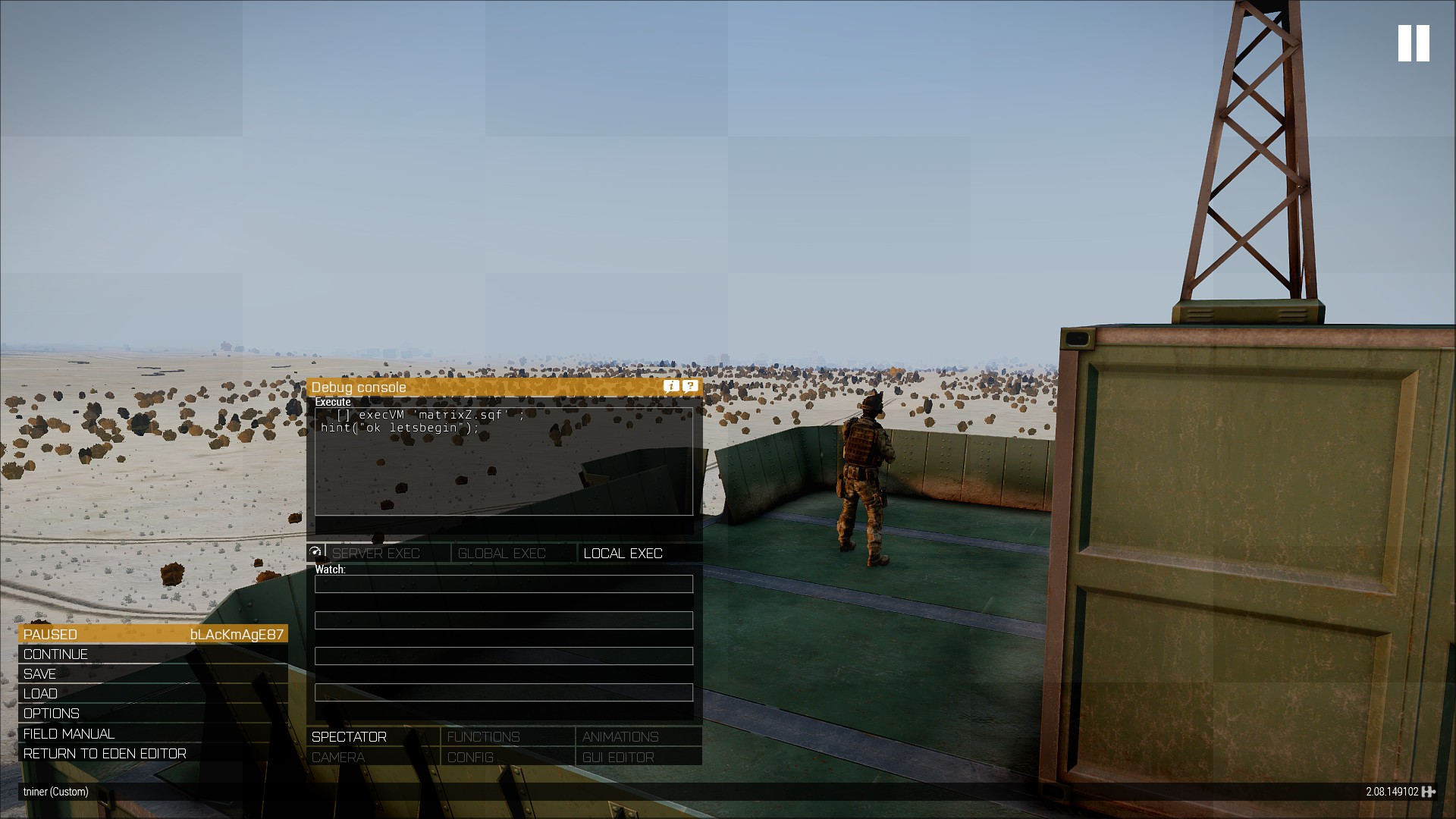Notice how long this takes... 2 mins plus... You should only have to do it once... after that you can keep the texts with the mods of your choice and quantity...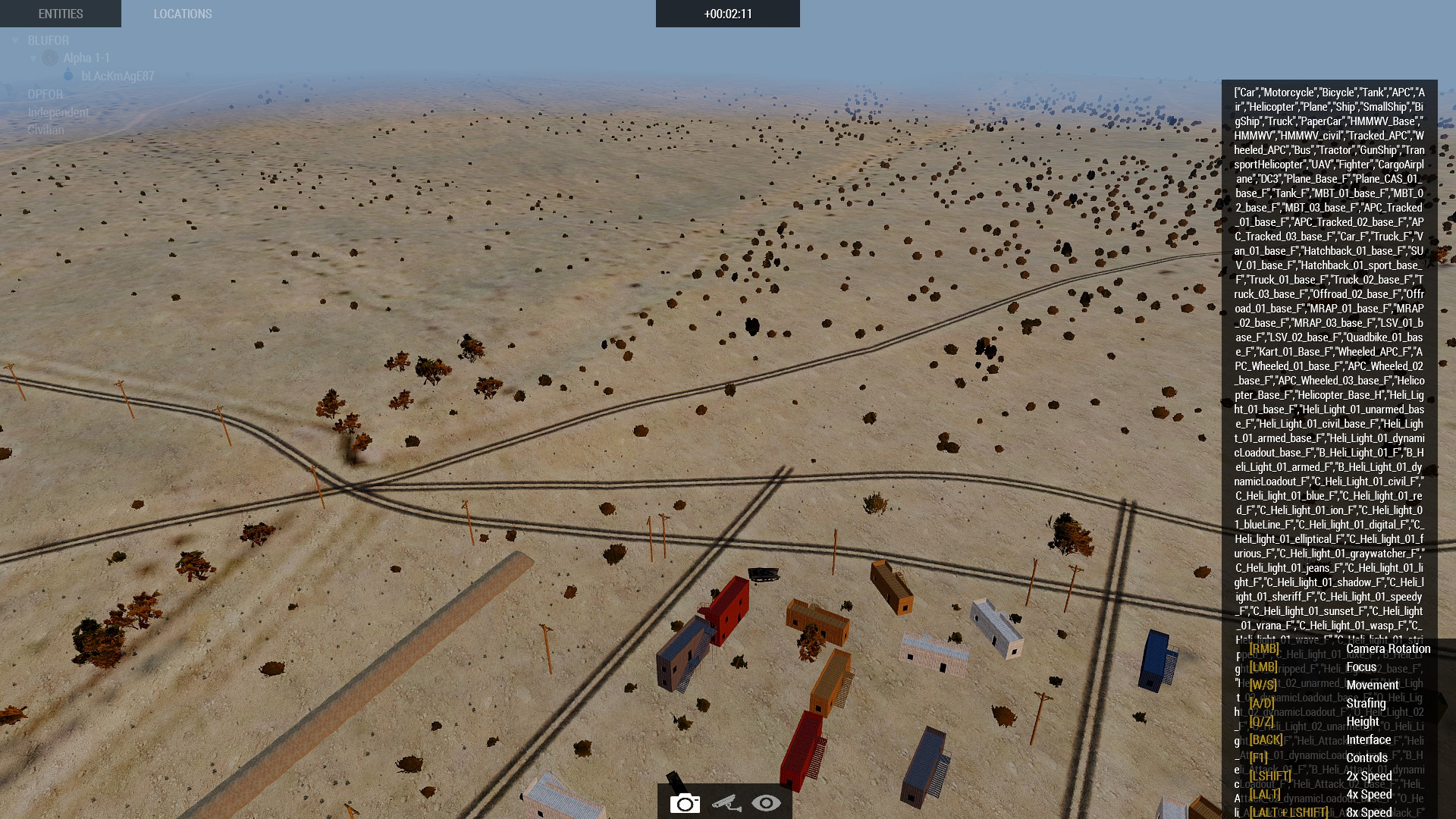Ok after I got the texts in place... I launch it for a fast  performance .... it only took a few seconds to start creating and finishes around 30s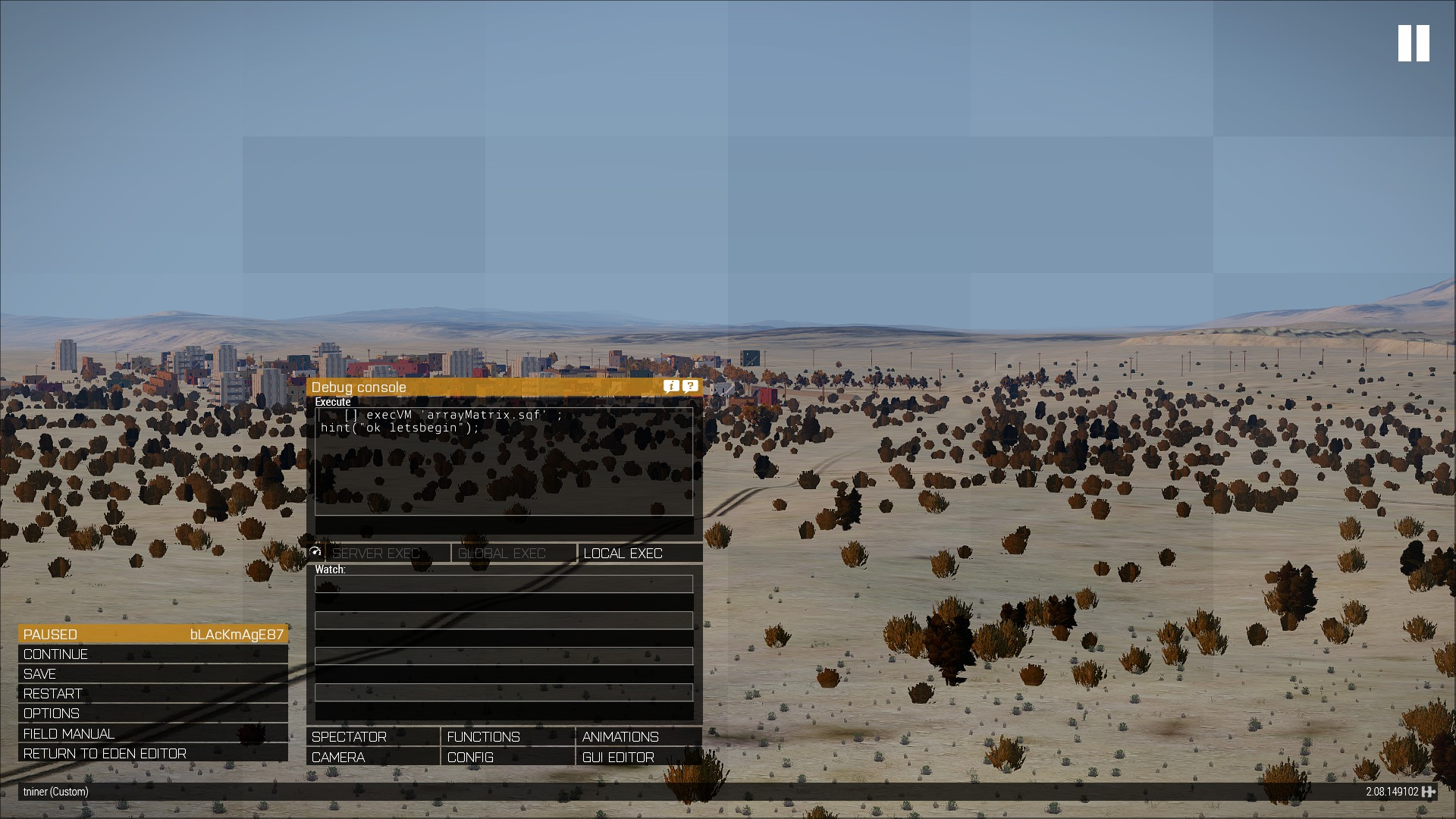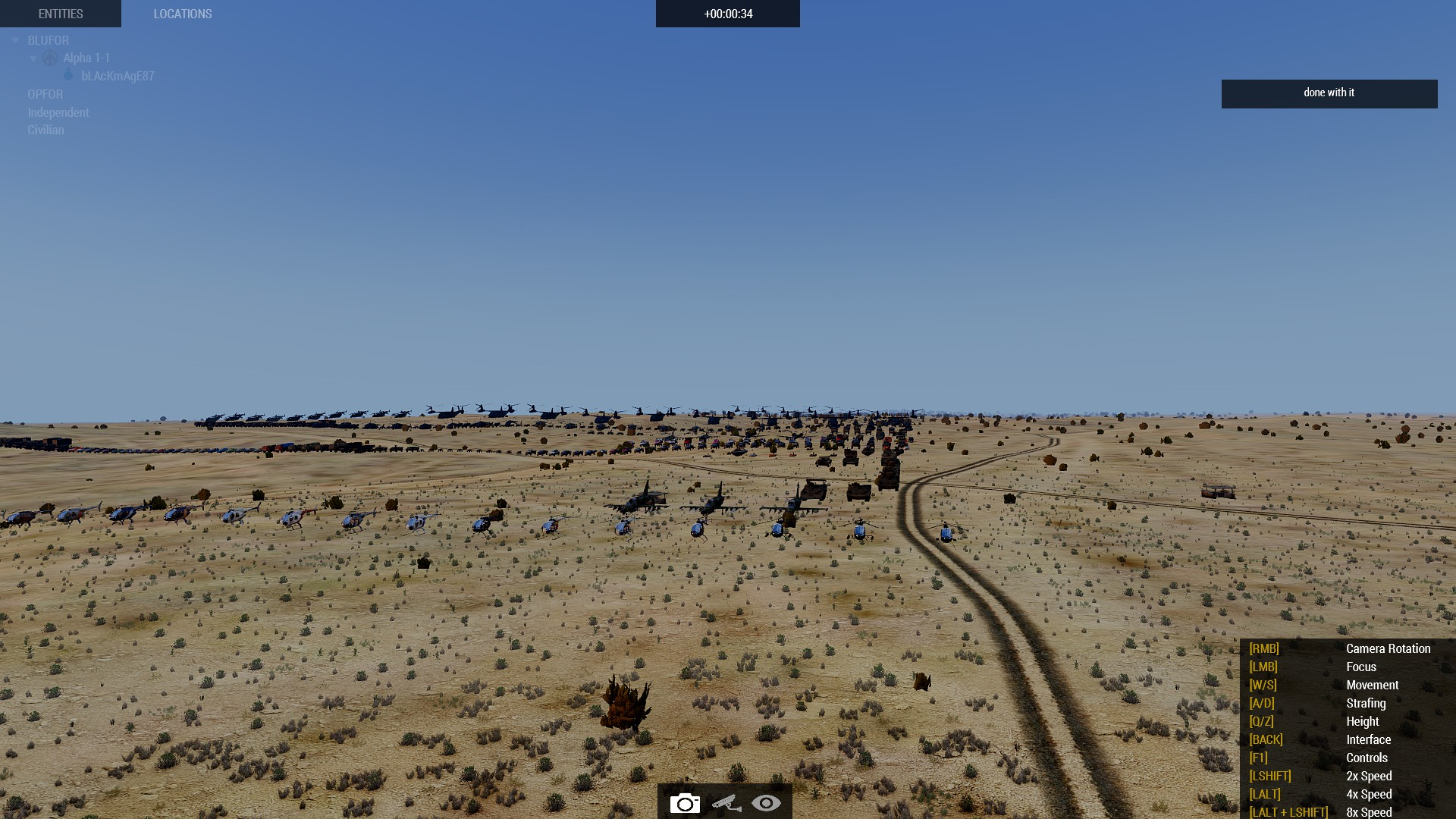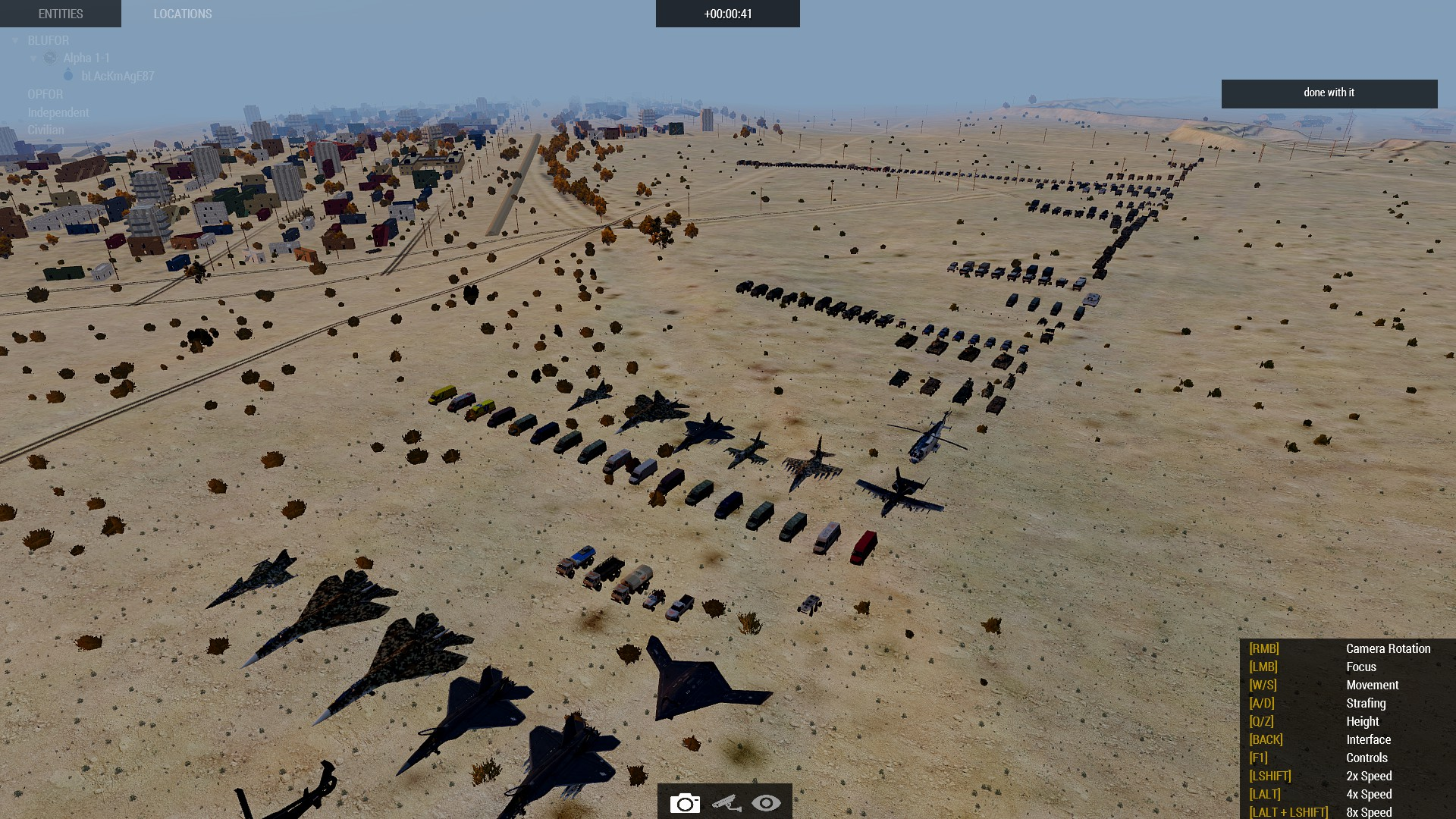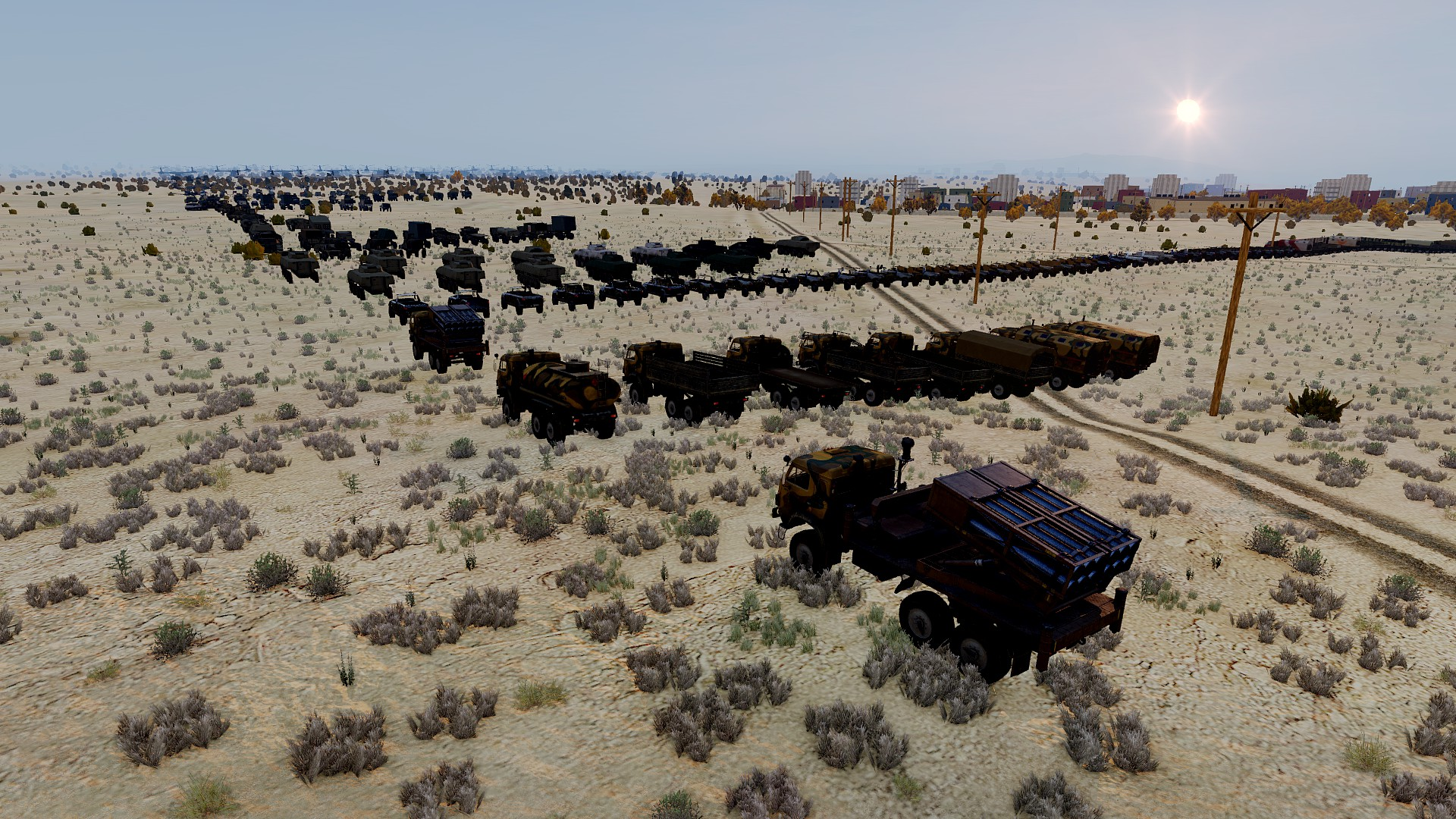Need markers and directional orientations